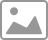# 我的 2018 Final 个人挑战（PART 2）：用深度学习为闹钟提供视觉能力

## 样本准备

``````import cv2
# 读取视频
rval, frame = vc.read()
# 视频帧计数间隔频率
duration = 10
# 循环读取视频
c = 1
while rval:
rval, frame = vc.read()
# 每隔10帧就保存照片
if(c%timeF == 0):
# 存储为图像
cv2.imwrite('smart_alarm/image/training/'occupied_' + str(c) + '.jpg', frame)
c = c + 1
cv2.waitKey(1)
``````

## 训练模型

``````import turicreate as tc

# 读取我在床上的图片，并打上标签：occupied
data = tc.image_analysis.load_images('/Users/patrick/CodeLab/alarm/image/training_occupied/', with_path=True)
data['label'] = 'occupied'
# 读取我不在床上的图片，并打上标签：empty
data_2 = tc.image_analysis.load_images('/Users/patrick/CodeLab/alarm/image/training_empty/', with_path=True)
data_2['label'] = 'empty'
# 合并到一个数据集里
data = data.join(data_2, how='outer')
``````

``````# 训练模型，选择 TuriCreate 里面的 image_classifier
model = tc.image_classifier.create(data, target='label', max_iterations=15)
``````

## 部署

``````# 保存到你的一个文件里
model.save('/Users/patrick/CodeLab/alarm/model')
``````

``````import base64
import turicreate as tc

# 读取模型

# 接口中要传输图像，可以使用 base64 的方式
def b64_to_file(data, filename):
'''
data: base64.b64encode(file)
filenmae: name of the file
'''
img_data = base64.b64decode(data)
path = '/root/codelab/alarm/tmp' + filename
try:
with open(path, 'wb') as f:
f.write(img_data)
return {
'result': 'success',
'path': path
}
except Exception as e:
return {
'result': 'fail',
'error': str(e)
}
def get_result(data, filename):
a = b64_to_file(data, filename)
if a['result'] == 'success':
prediction = model.predict(tc.Image(path=a['path']))
return {
'resultCode': '0000',
'prediction': prediction
}
else:
return {
'resultCode': '0001',
'error': a['error']
}
``````

``````from flask import Flask, jsonify
from flask import request
from flask import make_response
from flask import abort
import face_recognition as fr

# 这个就是接口地址
@app.route('/api/facerecognition/v1.0/checkface', methods=['GET', 'POST'])
def check_face():
if len(request.form['data']) < 10:
abort(404)
result = fr.get_result(request.form['data'], request.form['filename'])
return jsonify(result)

@app.errorhandler(404)
def not_found(error):

@app.errorhandler(400)
def missing_params(error):
return make_response(jsonify({'error': '参数缺失'}), 400)

if __name__ == '__main__':
app.run(host='0.0.0.0', port=80, debug=True)
``````

## 最后